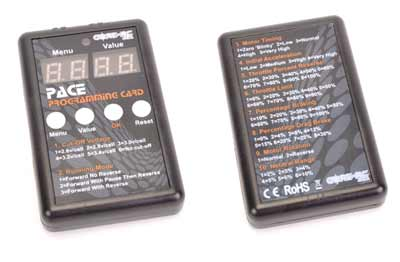# PACE ESC Program Card

### Part Number: SCH-CR174

£9.49 Web Price RRP £9.99

### Request Price Match

Optional program card available to allow multiple adjustments including;

1. Cut-Off Voltage
1=2.6v/cell  2=2.8v/cell  3=3.0v/cell 4=3.2v/cell  5=3.4v/cell  6=No cut-off
2. Running Mode
1=Forward No Reverse 2=Forward With Pause Then Reverse 3=Forward With Reverse
3. Motor Timing
1=Zero ‘Blinky’  2=Low  3=Normal 4=High   5=Very High
4. Initial Acceleration
1=Low  2=Medium  3=High 4=Very High
5. Throttle Percent Reverse
1=20%  2=30%  3=40% 4=50% 5=60% 6=70%  7=80%  8=90% 9=100%
6. Throttle Limit
1=0%  2=20%  3=30% 4=40% 5=50% 6=60%  7=70%  8=80% 9=90%
7. Percentage Braking
1=10%  2=20%  3=30% 4=40% 5=50% 6=60%  7=70%  8=80% 9=100%
8. Percentage Drag Brake
1=0%  2=4%  3=8% 4=12% 5=15% 6=20%  7=25%  8=30%
9. Motor Rotation
1=Normal  2=Reverse
10. Neutral Range
1=2%  2=3%  3=4% 4=5% 5=6%  6=10%

## Two Simple UK Delivery Options

Royal mail at £3.50, or Express at £6.95.

Full details at checkout.
Some items cannot be sent via Royal Mail due to their restrictions.

### We Ship Worldwide!

To see shipping costs simply add the item to your basket and choose your country from the drop down list on the view cart page.

No reviews yet!
No questions yet, why not ask one?